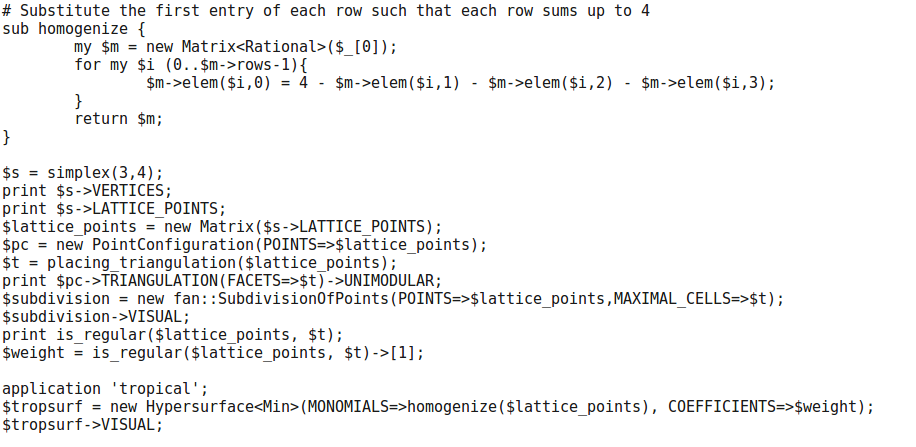# Problem 13¶

Draw a unimodular triangulation of 4 times the unit tetrahedron. Draw the smooth tropical quartic surface that is dual to your triangulation.

## Solution¶

In Polymake:

Substitute the first entry of each row such that each row sums up to 4

```sub homogenize {
my \$m = new Matrix<Rational>(\$_);
for my \$i (0..\$m->rows-1){
\$m->elem(\$i,0) = 4 - \$m->elem(\$i,1) - \$m->elem(\$i,2) - \$m->elem(\$i,3);
}
return \$m;
}

\$s = simplex(3,4);
print \$s->VERTICES;
print \$s->LATTICE_POINTS;
\$lattice_points = new Matrix(\$s->LATTICE_POINTS);
\$pc = new PointConfiguration(POINTS=>\$lattice_points);
\$t = placing_triangulation(\$lattice_points);
print \$pc->TRIANGULATION(FACETS=>\$t)->UNIMODULAR;
\$subdivision = new fan::SubdivisionOfPoints(POINTS=>\$lattice_points,MAXIMAL_CELLS=>\$t);
\$subdivision->VISUAL;
print is_regular(\$lattice_points, \$t);
\$weight = is_regular(\$lattice_points, \$t)->;

application 'tropical';
\$tropsurf = new Hypersurface<Min>(MONOMIALS=>homogenize(\$lattice_points), COEFFICIENTS=>\$weight);
\$tropsurf->VISUAL;
```